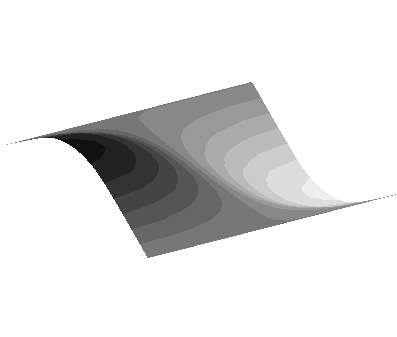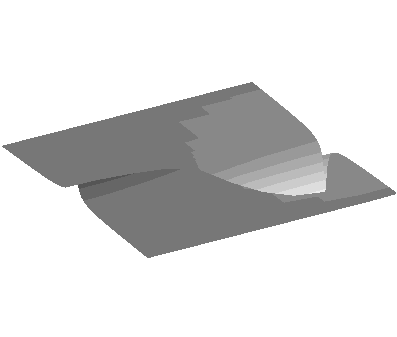研究成果・発表論文

Self-Similar Viscous Collapse of a Self-Gravitating, Polytropic Gas Disk

Mineshige, Shin,   Nakayama, Kunji,   & Umemura, Masayuki

要旨
Self-similar solutions for the time evolution of polytropic, self- gravitating viscous disks is found on the condition that the kinematic viscosity is nu åisebox-0.5ex~ r(\l\ and the gas pressure is P \i̊sebox-0.5ex~ rho (Gamma ) , where l and Gamma are free parameters, r is radius, and rho is density. This solution describes a self-similar collapse of a rotating gas disk via self-gravity and viscosity. For Gamma < Gc = (3+2l)/(3+l) global accretion solutions exist, provided that 0 < l <= 1. Owing to the boundary condition of no radial velocity at the origin, mass is accumulated near to the center, giving rise to a strong central concentration; the surface density varies as Sigma \rs̊ebox-0.5ex~ r(-1-(2)/(3)l) . In the outer portions, in contrast, the surface density more slowly decreases outward as Sigma \rae̊box-0.5ex~ r(-Gamma /(2-Gamma )) . For Gamma > Gc , conversely, no global accretion solution is found.